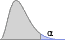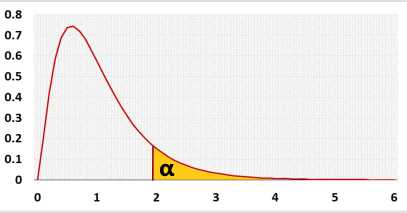# F Statistical power calculatorPower analysis
Legend (right tail example)

H0:H1:## Information

Calculate the test power basted on the sample size and draw a power analysis chart.
Use this calculator for the following test:
F test For variances calculator

### F Distribution## R Code

The following R code should produce the same results